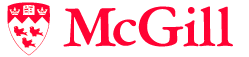# COMP/MATH 553: Algorithmic Game Theory

## Course Outline

• Introduction
• Games, solution concepts, auctions.
• Nash's theorem
• Von Neumann's Minimax theorem;
• Algorithms for computing Nash equilibria: support enumeration algorithms, symmetries, the Lemke Howson algorithm, sampling methods;
• Mechanism Design
• Social Choice Theory: Arrow's impossibility theorem, the Gibbard-Satterthwaite theorem;
• Second-price auction, sponsored search auction;
• Myerson's Lemma: characterization of single-parameter incentive compatible mechanisms, revenue equivalence;
• Myerson's Optimal Auction;
• The Revelation Principle;
• Prior-independent mechanisms: prophet inequality, Bulow-Klemperer;
• Vickrey-Clarke-Groves mechanism, spectrum auctions;
• Multi-dimensional Mechanism Design;
• Stable Matching, Kidney Exchange
• The Complexity of Computing Nash Equilibria
• Nash's Theorem: proof via Sperner's lemma; emphasis on the combinatorial proofs of existence;
• Complexity theory of total search problems and fixed points: definition of the complexity classes PLS, PPAD, PPP and relation to P, NP;
• The complexity of computing a Nash equilibrium: PPAD-completeness results;
• The complexity of pure Nash equilibria in congestion games: PLS-completeness results;
• Other Solution Concepts
• Correlated Equilibria, Algorithm: ellipsoid against hope;
• Game Dynamics: fictitious play;
• No-regret Learning: multiplicative weights update method, Coarse Correlated Equilibria;
• Market Equilibria
• The Arrow-Debreu existence theorem;
• Algorithms for markets with linear utility functions;
• Tatonnement;
• PPAD-hardness of markets with Leontief utility functions.
• Price of Anarchy: selfish routing in networks, PoA in congestion games.